# Write DQ/DBI Per-bit Deskew and Centering (Simple) - 1.0 English

Document ID
PG313
Release Date
2023-11-01
Version
1.0 English

## Write Per-Bit DQ Deskew

Initially all the DQ bits associated with a DQS strobe have the same ODELAY setting based on the write leveling results, but the ODELAY for each bit might need to be adjusted to account for skew between bits. The following figure shows an example of the initial timing relationship between a write DQS and DQ.

Figure 1. Initial Write DQS and DQ with Skew Between Bits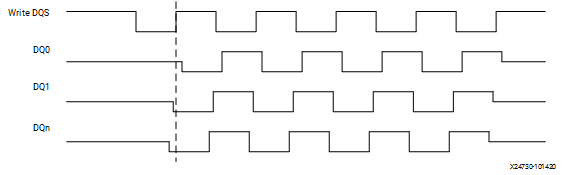1. Turn off DBI/DM on the write path and DBI on the read path (MRS setting in the DRAM and fabric switch).
2. Write pattern `10101010` into the DRAM memory and read back. Here, MSB is the last bit of a burst and LSB is the first bit of a burst. The data read back on some DQ bits are `10101010` while other DQ bits might be `01010101` due to skew between the DQ bits.
3. Increment DQS ODELAY one fine tap at a time (maximum shift limited to 90⁰) for finding common valid window (15 taps wide) for all DQ bits. This step is carried out in parallel for all DQS. Once valid window is detected for a DQ bit, increment ODELAY for that DQ bit along with DQS until common valid window is detected for the remaining DQ bits (see the following figure). At the end of this step, common valid window detection status of write DQS is saved as BRAM_WRDQDBI_STG1_BYTE_STATUS* and DQ bits for which valid window is detected is saved as BRAM_WRDQDBI_STG1_BIT_STATUS*.
Figure 2. Increment Write DQS ODELAY Until All Bits Captured with Correct Pattern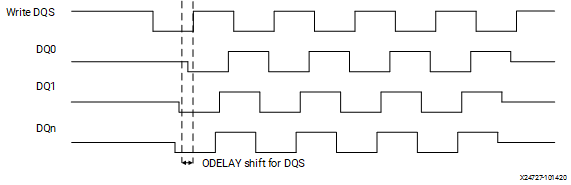4. If a DQS is unable to detect common valid window before reaching maximum shift of 90° (see the following figure), ODELAY for DQS and DQ (bits for which valid window is detected) are reverted back to point where last valid window is detected for a DQ bit. At the end of this step, DQS ODELAY value is saved as BRAM_WRDQDBI_STG2_DQS_ODLY_BYTE* and DQ bits ODELAY value is saved as BRAM_WRDQDBI_STG2_DQ_ODLY_BIT*.
Figure 3. Write DQS ODELAY Reached 90° Limit While Searching for Correct Pattern for All DQ Bits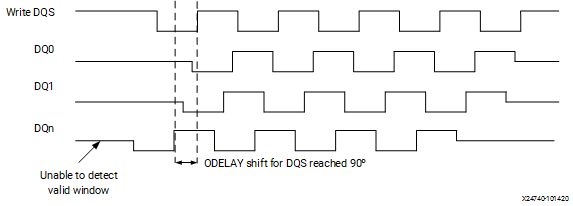5. ODELAY value of DQS for which common valid window is detected for at least one of the DQ bits is reverted back by minimum window width of 15 taps. Increment DQ ODELAY one tap at a time (maximum shift limited to 180⁰) for detecting common valid window on remaining DQ bits (see the following figure). At the end of this step, DQS ODELAY value is saved as BRAM_WRDQDBI_STG1_DQS_DELAY_BYTE* and DQ bits ODELAY value is saved as BRAM_WRDQDBI_STG3_DQ_ODLY_BIT*.
Figure 4. Increment DQ ODELAY until All Bits Captured with Correct Pattern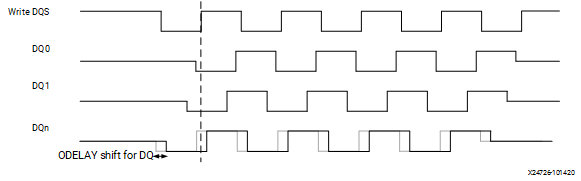6. Increment each DQ ODELAY using step size of 10 taps until each bit fails to return the expected data pattern. Once completed, decrement each DQ ODELAY using step size of 10 taps. At the end of this step, DQ ODELAY value is saved as BRAM_WRDQDBI_DESKEW_DQ_ODLY_FCRSE_BIT*.
7. Increment each DQ ODELAY by one fine tap at a time until each bit fails to return the expected data pattern (the data is edge aligned with the write DQS, see the following figure). At the end of this step, DQ ODELAY value is saved as BRAM_WRDQDBI_DESKEW_DQ_ODLY_BIT*.
Figure 5. DQ Per-Bit Write Deskew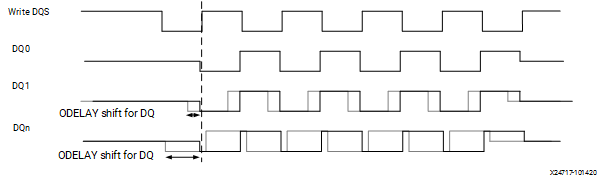## CAL_ERROR Decode for Write Per-Bit DQ Deskew Calibration

The status of Write Per-Bit DQ Deskew can also be determined by decoding the CAL_ERROR result according to the following table.

Table 1. CAL_ERROR Decode for Write Per-Bit DQ Deskew Calibration
Error Code Description Recommended Debug Step
0x15 No valid data found for a given bit in the byte by incrementing DQS ODELAY first and then DQ ODELAY. Check the alignment of DQS to DQ during a write burst with a scope on the PCB. Check the DQS-to-CK alignment. Check the WRLVL fields in XSDB for a given byte.
0x16 Noise region not found for a given bit in the byte by incrementing DQ ODELAY using step size of 10 taps. Check for a mapping issue. This usually implies a delay is not moving when it should. Check the connections going to the XPHY and ensure the correct RIU is selected based on the byte being adjusted.
0x17 Noise region not found for a given bit in the byte by incrementing DQ ODELAY using step size of 1 tap. Check for a mapping issue. This usually implies a delay is not moving when it should. Check the connections going to the XPHY and ensure the correct RIU is selected based on the byte being adjusted.

## Write Per-Bit DBI Deskew

Note: The calibration step is only enabled for the first rank in a multi-rank system.

Initially DBI and DQS strobe have the same ODELAY setting based on the write leveling results, but ODELAY values of DQS are modified during write DQ per-deskew. A similar mechanism as the write DQ per-bit deskew is run but DBI pin is deskewed instead in relation to DQS. The following figure shows an example of the initial timing relationship between a write DQS and DBI.

1. Turn on DBI on the write path, turn off DM on the write path, and DBI on the read path (MRS setting in the DRAM and fabric switch).
2. Write pattern `10101010` into the DRAM memory (extending the data pattern before/after the burst because write latency calibration has not yet been performed) and read back the data. The data read back on some DQ bytes might be different from the expected pattern `FF00FF00_FF00FF00` due to skew between DBI and DQS.
Figure 6. Initial Write DQS and DBI with Skew Between Them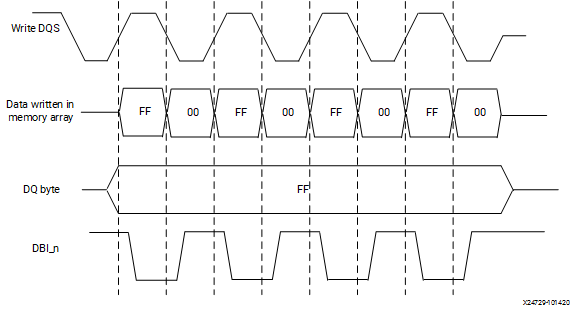3. Increment DQS ODELAY by one fine tap at a time (maximum shift limited to 90°) for finding valid window (15 taps wide) for the data read on DQ byte (see the following figure). This step is carried out in parallel for all DQS. At the end of this step, valid window detection status is saved as BRAM_WRDQDBI_STG4_BYTE_STATUS*.
Figure 7. Increment Write DQS ODELAY Until DQ Byte Capture Correct Pattern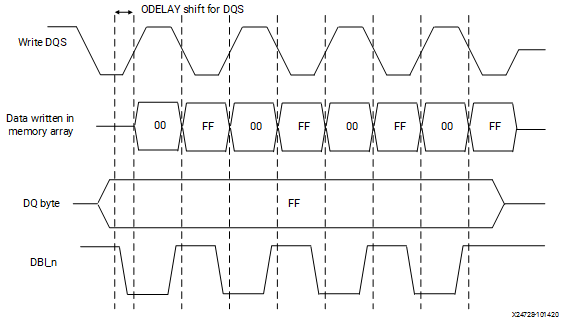4. If a DQS is unable to detect valid window before reaching maximum shift of 90° (see the following figure), ODELAY for that DQS is reverted to initial value. At the end of this step, DQS ODELAY value is saved as BRAM_WRDQDBI_STG5_DQS_ODLY_BYTE*.
Figure 8. Write DQS ODELAY Reached 90° Limit While Searching for Correct Pattern for DQ Byte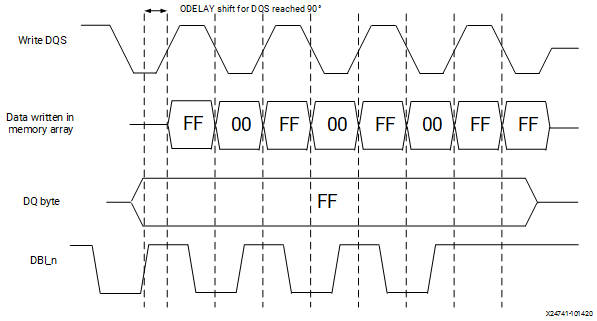5. ODELAY value of DQS for which valid window is detected is reverted back by minimum window width of 15 taps. Increment DBI ODELAY by one fine tap at a time (maximum shift limited to 180°) for detecting valid window of remaining DQS strobe (see the following figure). At the end of this step, DQS ODELAY value is saved as BRAM_WRDQDBI_STG4_DQS_DELAY_BYTE* and DBI ODELAY value is saved as BRAM_WRDQDBI_STG6_DBI_ODLY_BYTE*.
Figure 9. Increment DBI ODELAY Until DQ Byte Capture Correct Pattern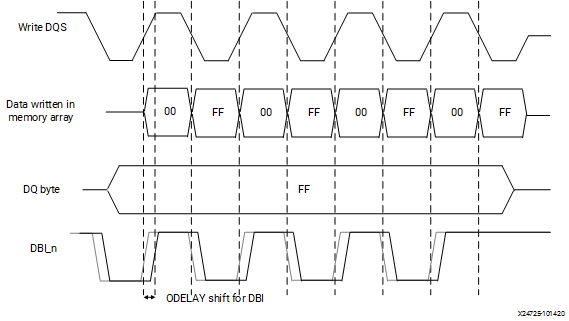6. Increment each DBI ODELAY using step size of 10 taps until DQ byte fails to return the expected data pattern. Once completed, decrement each DBI ODELAY by step size of 10 taps. At the end of this stage, DBI ODELAY values are saved as BRAM_WRDQDBI_DESKEW_DBI_ODLY_FCRSE_BYTE*.
7. Increment each DBI ODELAY by one fine tap at a time until DQ byte fails to return the expected data pattern (DBI is edge aligned with write DQS, see figure below). At the end of this step, DBI ODELAY values are stored as BRAM_WRDQDBI_DESKEW_DBI_ODLY_BYTE*.
Figure 10. Write DBI Bit Deskew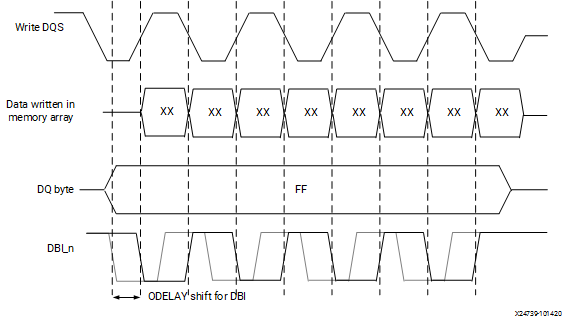## CAL_ERROR Decode for Write Per-Bit DBI Deskew Calibration

The status of Write Per-Bit DBI Deskew can also be determined by decoding the CAL_ERROR result according to the following table.

Table 2. CAL_ERROR Decode for Write Per-Bit DBI Deskew Calibration
Error Code Description Recommended Debug Step
0x18 No valid data found for a given bit in the byte by incrementing DQS ODELAY first and then DBIODELAY. Check the alignment of DQS to DBI during a write burst with a scope on the PCB. Check the DQS-to-CK alignment. Check the WRLVL fields in XSDB for a given byte.
0x19 Noise region not found for a given bit in the byte by incrementing DBI ODELAY using step size of 10 taps. Check for a mapping issue. This usually implies a delay is not moving when it should. Check the connections going to the XPHY and ensure the correct RIU is selected based on the byte being adjusted.
0x1A Noise region not found for a given bit in the byte by incrementing DBI ODELAY using step size of 1tap. Check for a mapping issue. This usually implies a delay is not moving when it should. Check the connections going to the XPHY and ensure the correct RIU is selected based on the byte being adjusted.

## Write DQS to DQ/DBI Centering

After write DQ per-bit and DBI bit deskew, the next step is to determine the relative center of the DQS in the write data eye.

1. Left margin of write data for a DQS is calculated as the largest value among DQ bits delay and DBI delay calculated during deskew. ODELAY of DQ bits and DBI is decremented by step size equal to the left margin. At the end of this step, DQ ODELAY value is saved as BRAM_WRDQDBI_LEFT_EDGE_DQ_BIT* and final ODELAY values for DBI are saved as BRAM_WRDQDBI_LEFT_EDGE_DBI_BYTE*.
2. Issue a set of write and read bursts with the data pattern `10101010` and check the read data. Just as in write DQ per-bit deskew when issuing a write to the DRAM, the DQS and DQ toggles for eight clock cycles before and after the expected write latency. This is used to ensure the data is written into the DRAM even if the command-to-write data relationship is still unknown.
3. Find the right edge of the write data window by incrementing DQS ODELAY by step size of 10 taps until the data changes from the expected data pattern `10101010`. Once completed, decrement each DQS ODELAY by step size of 10 taps. At the end of this stage, right margin values are saved as BRAM_WRDQDBI_RIGHT_MARGIN_FCRSE_BYTE*.
4. Increment each DQS ODELAY by one fine tap at a time until the data changes from the expected data pattern `10101010` (see the following figure). At the end of this step, right margin values are saved as BRAM_WRDQDBI_RIGHT_MARGIN_BYTE*.
Figure 11. Write DQS to DQ/DBI Centering – Right Margin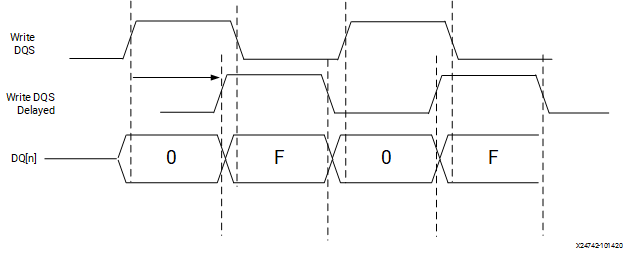5. Calculate the center tap location for the DQS ODELAY, based on left margin and right margin. Final DQS, DQ, and DBI ODELAY values are calculates as:
• Right Margin > Left Margin
• DQS ODELAY = initial ODELAY - [(Left Margin + Right Margin) / 2
• DQ ODELAY = No change
• DBI ODELAY = No change
• Left Margin > Right Margin
• DQS ODELAY = No change
• DQ ODELAY = initial ODELAY + [(Left Margin - Right Margin) / 2]
• DBI ODELAY = initial ODELAY + [(Left Margin - Right Margin) / 2]

The final ODELAY tap settings for DQS, DQ, and DBI are indicated by BRAM_WRDQDBI_ODLY_DQS_FINAL_BYTE*, BRAM_WRDQDBI_ODLY_DQ_FINAL_BIT*, and BRAM_WRDQDBI_ODLY_DBI_FINAL_BYTE*, respectively.

## CAL_ERROR Decode for Write DQS to DQ/DBI Centering Calibration

The status of Write DQS to DQ/DBI Centering can also be determined by decoding the CAL_ERROR result according to the following table.

Table 3. CAL_ERROR Decode for Write DQS to DQ/DBI Centering Calibration
Error Code Description Recommended Debug Step
0x1B Right edge of write data not found by incrementing DQS ODELAY using step size of 10 taps. Check for a mapping issue. This usually implies a delay is not moving when it should. Check the connections going to the XPHY and ensure the correct RIU is selected based on the byte being adjusted.
0x1C Right edge of write data not found by incrementing DQS ODELAY using step size of 1 tap. Check for a mapping issue. This usually implies a delay is not moving when it should. Check the connections going to the XPHY and ensure the correct RIU is selected based on the byte being adjusted.
0x1D Post sanity check failed. Check CAL_ERROR_BIT_*_*,CAL_ERROR_DATA_NIBBLE_*_*,CAL_ERROR_PHY_NIBBLE_*_* XSDB registers to determine which nibbles/bits failed. Check margin found during previous stages of calibration for the given byte that failed.
Table 4. Write DQ/DBI Per-bit Deskew and Centering (Simple) Registers
Register Name Quantity Description
Fx_WRDQDBI_STG1_DQS_DELAY Byte DQS odelay after finding valid window by moving DQS.
Fx_WRDQDBI_STG1_BYTE_STATUS 2 Value of 1 if a valid window is found. One bit per byte lane.
Fx_WRDQDBI_STG1_BIT_STATUS DBI pins Value of 1 if a valid window is found.
Fx_WRDQDBI_STG2_DQS_ODLY Byte Odelay for DQS after reverting.
Fx_WRDQDBI_STG2_DQ_ODLY DQ bit Odelay for DQ after reverting.
Fx_WRDQDBI_STG3_DQ_ODLY DQ bit Odelay value for the valid window using DQ delay.
Fx_WRDQDBI_DESKEW_DQ_ODLY_FCRSE DQ bit Deskew delay 10-tap increments.
Fx_WRDQDBI_DESKEW_DQ_ODLY DQ bit Deskew delay.
Fx_WRDQDBI_STG4_DQS_DELAY DBI pin DQS Odelay value for the valid window.
Fx_WRDQDBI_STG4_BYTE_STATUS 2 1 if a valid window is found. 1 bit per byte lane.
Fx_WRDQDBI_STG5_DQS_ODLY DBI pin Odelay after reverting DQS.
Fx_WRDQDBI_STG6_DBI_ODLY DBI pin DBI Odelay value for valid window using.
Fx_WRDQDBI_DESKEW_DBI_ODLY_FCRSE DBI pin Deskew delay 10-tap increments.
Fx_WRDQDBI_DESKEW_DBI_ODLY DBI pin Deskew delay.
Fx_WRDQDBI_LEFT_MARGIN Byte Smallest margin left of center for the byte in Odelay taps.
Fx_WRDQDBI_LEFT_EDGE_DQ DQ bit Odelay value for left edge of DQ.
Fx_WRDQDBI_LEFT_EDGE_DBI DBI bit Odelay value for left edge of DBI.
Fx_WRDQDBI_RIGHT_MARGIN_FCRSE Byte Right margin DQS Odelay with 10-tap increments.
Fx_WRDQDBI_RIGHT_MARGIN Byte Smallest margin right of center for the byte in Odelay taps.
Fx_WRDQDBI_RIGHT_EDGE_DQS Byte DQS Odelay value for the right edge.
Fx_WRDQDBI_ODLY_DQS_FINAL Byte Final DQS Odelay value.
Fx_WRDQDBI_ODLY_DQ_FINAL DQ bit Final DQ Odelay value.
Fx_WRDQDBI_ODLY_DBI_FINAL DBI bit Final DBI Odelay value.